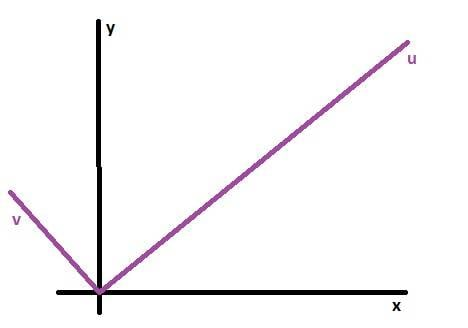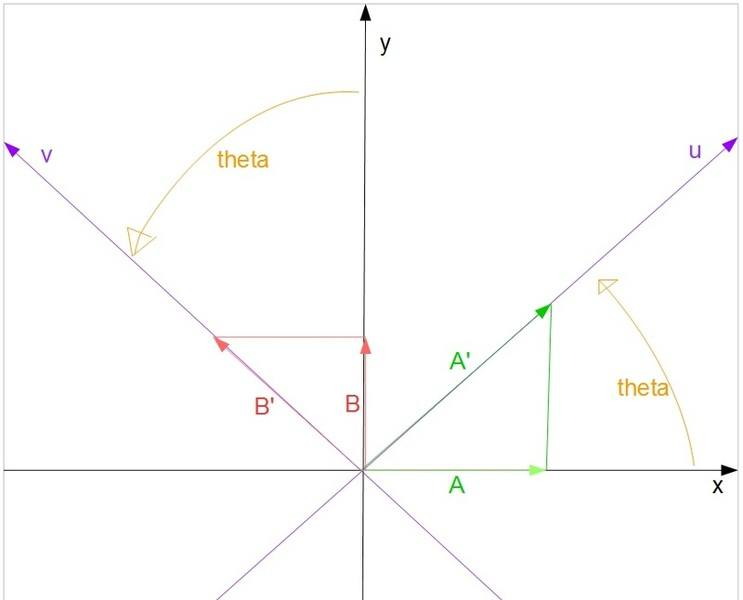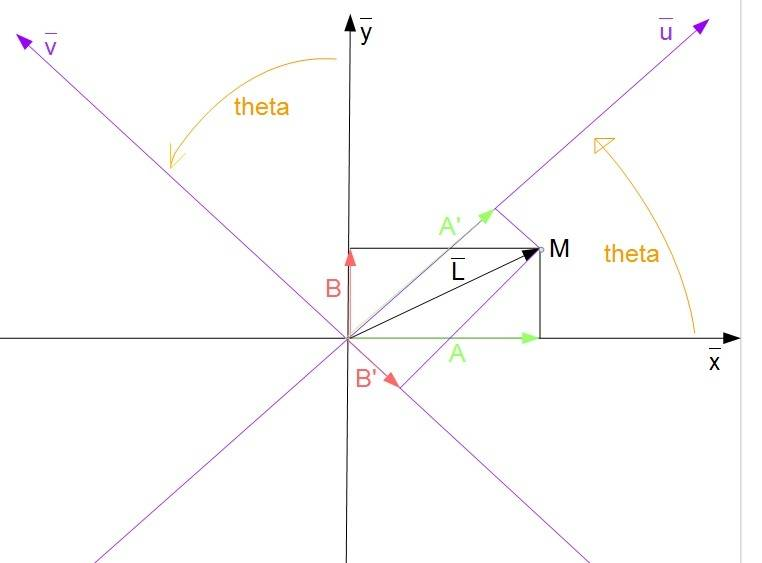# Deriving maximum velocity in a rotated frame

• II have a particle that travels in the cartesian plane with the maximum velocity of A units along the x-axis and B units along the y-axis per unit of time.

How do I go about deriving the maximum velocity of my particle in the rotated uv plane? (the maximum distance the particle can along the u and the v axes in one unit of time)

Any tips greatly appreciated.

Buzz Bloom
Gold Member
Hi jumbo:

I suggest it might help if you add an arrow in the diagram to represent the vector M from the origin to point (x=A,y=B). Then draw perpendiculars from (A,B) to the axes u and v. If you know the angle between x axis and u axis, you can calculate the length of the perpendiculars to the u axis and v axis which represent the M coordinates with respect to the v axis and u axis respectively.

Hope this helps.

Regards,
Buzz

Thanks Buzz Bloom.

Is this what you mean?Where the length of A' is the maximum distance my particle can travel along the u-axis of the rotated frame in one unit of time. Similarly, the length of B'...

Last edited:
Buzz Bloom
Gold Member
Hi jumbo:

I apologize for not expressing my thought more clearly.

You want to draw a line, call it L, from the origin to the point M. M will be on the vertical green line at the height B. L represents the vector corresponding to the maximum velocity of the particle you are describing.

Next draw lines from the point M perpendicular to the u and v axes. These lines will show the coordinates of the vector L in the (u,v) coordinate system. The resulting diagram should help you figure out how to calculate the u and v coordinates of the vector L.

By the way, for the particular example you have drawn, you may want to notice that the v coordinate of L is negative.

Hope this helps.

Regards,
Buzz

I think I got it. Thanks Buzz•Buzz Bloom